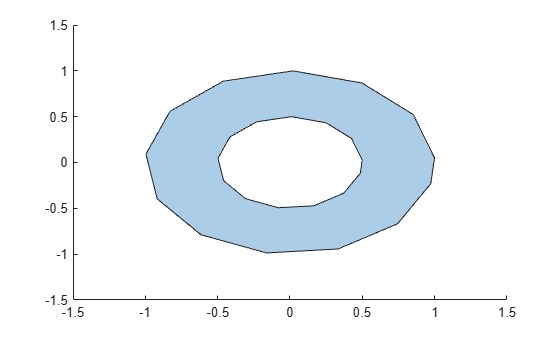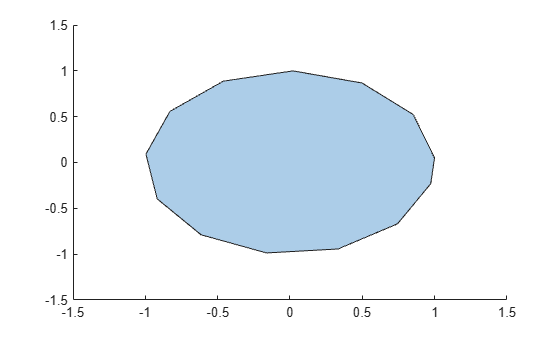rmholes

Remove holes in polyshape

Description

example

polyout = rmholes(polyin) returns a polyshape object made up of the solid boundaries of the polygon polyin, with all the hole boundaries removed.

Examples

collapse all

Create a polygon with a hole.

t = 0.05:0.5:2*pi;
x1 = cos(t);
y1 = sin(t);
x2 = 0.5*cos(t);
y2 = 0.5*sin(t);
polyin = polyshape({x1,x2},{y1,y2})
polyin =
polyshape with properties:

Vertices: [27x2 double]
NumRegions: 1
NumHoles: 1

plot(polyin)Create a new polygon with the hole removed.

polyout = rmholes(polyin)
polyout =
polyshape with properties:

Vertices: [13x2 double]
NumRegions: 1
NumHoles: 0

plot(polyout)Input Arguments

collapse all

Input polyshape, specified as a scalar, vector, matrix, or multidimensional array.

Data Types: polyshape